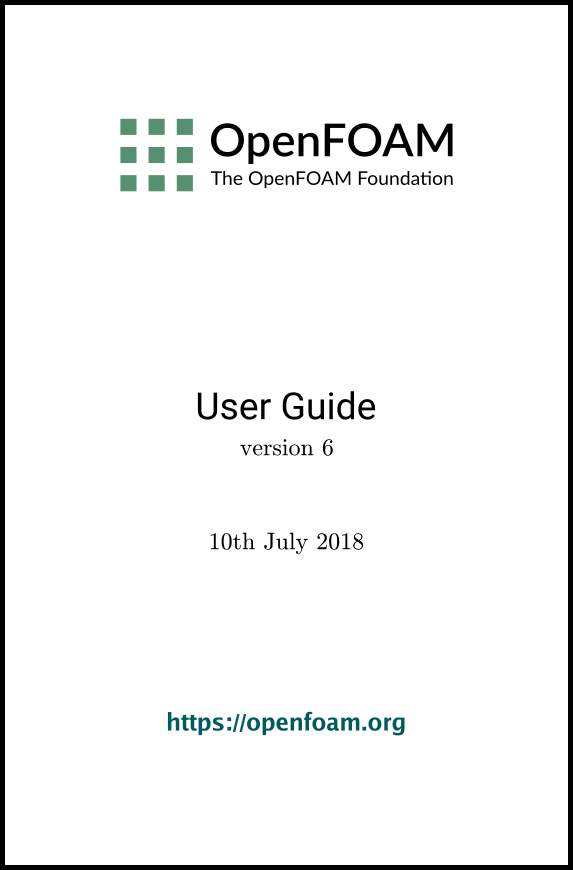## 7.2 Turbulence models

The turbulenceProperties dictionary is read by any solver that includes turbulence modelling. Within that file is the simulationType keyword that controls the type of turbulence modelling to be used, either:

laminar
uses no turbulence models;
RAS
uses Reynolds-averaged simulation (RAS) modelling;
LES
uses large-eddy simulation (LES) modelling.

### 7.2.1 Reynolds-averaged simulation (RAS) modelling

If RAS is selected, the choice of RAS modelling is specified in a RAS sub-dictionary which requires the following entries.

• RASModel: name of RAS turbulence model.
• turbulence: switch to turn the solving of turbulence modelling on/off.
• printCoeffs: switch to print model coeffs to terminal at simulation start up.
• <RASModel>Coeffs: optional dictionary of coefficients for the respective RASModel, to override the default coefficients.

Turbulence models can be listed by running a solver with the -listTurbulenceModels option, e.g.

simpleFoam -listTurbulenceModels
With simpleFoam, the incompressible models are listed. The compressible models are listed for a compressible solver, e.g. rhoSimpleFoam.

The RAS models used in the tutorials can be listed using foamSearch with the following command. The lists of available models are given in the following sections.

foamSearch \$FOAM_TUTORIALS RAS.RASModel turbulenceProperties
Users can locate tutorials using a particular model, e.g. buoyantKEpsilon, using foamInfo.

foamInfo buoyantKEpsilon

#### 7.2.1.1 Incompressible RAS turbulence models

For incompressible flows, the RASModel can be chosen from the list below.

LRR
Launder, Reece and Rodi Reynolds-stress turbulence model for incompressible flows.
LamBremhorstKE
Lam and Bremhorst low-Reynolds number k-epsilon turbulence model for incompressible flows.
LaunderSharmaKE
Launder and Sharma low-Reynolds k-epsilon turbulence model for incompressible flows.
LienCubicKE
Lien cubic non-linear low-Reynolds k-epsilon turbulence models for incompressible flows.
LienLeschziner
Lien and Leschziner low-Reynolds number k-epsilon turbulence model for incompressible flows.
RNGkEpsilon
Renormalization group k-epsilon turbulence model for incompressible flows.
SSG
Speziale, Sarkar and Gatski Reynolds-stress turbulence model for incompressible flows.
Shih’s quadratic algebraic Reynolds stress k-epsilon turbulence model for incompressible flows
SpalartAllmaras
Spalart-Allmaras one-eqn mixing-length model for incompressible external flows.
kEpsilon
Standard k-epsilon turbulence model for incompressible flows.
kOmega
Standard high Reynolds-number k-omega turbulence model for incompressible flows.
kOmegaSST
Implementation of the k-omega-SST turbulence model for incompressible flows.
kOmegaSSTLM
Langtry-Menter 4-equation transitional SST model based on the k-omega-SST RAS model.
kOmegaSSTSAS
Scale-adaptive URAS model based on the k-omega-SST RAS model.
kkLOmega
Low Reynolds-number k-kl-omega turbulence model for incompressible flows.
qZeta
Gibson and Dafa’Alla’s q-zeta two-equation low-Re turbulence model for incompressible flows
realizableKE
Realizable k-epsilon turbulence model for incompressible flows.
v2f
Lien and Kalitzin’s v2-f turbulence model for incompressible flows, with a limit imposed on the turbulent viscosity given by Davidson et al.

#### 7.2.1.2 Compressible RAS turbulence models

For compressible flows, the RASModel can be chosen from the list below.

LRR
Launder, Reece and Rodi Reynolds-stress turbulence model for compressible flows.
LaunderSharmaKE
Launder and Sharma low-Reynolds k-epsilon turbulence model for compressible and combusting flows including rapid distortion theory (RDT) based compression term.
RNGkEpsilon
Renormalization group k-epsilon turbulence model for compressible flows.
SSG
Speziale, Sarkar and Gatski Reynolds-stress turbulence model for compressible flows.
SpalartAllmaras
Spalart-Allmaras one-eqn mixing-length model for compressible external flows.
buoyantKEpsilon
Additional buoyancy generation/dissipation term applied to the k and epsilon equations of the standard k-epsilon model.
kEpsilon
Standard k-epsilon turbulence model for compressible flows including rapid distortion theory (RDT) based compression term.
kOmega
Standard high Reynolds-number k-omega turbulence model for compressible flows.
kOmegaSST
Implementation of the k-omega-SST turbulence model for compressible flows.
kOmegaSSTLM
Langtry-Menter 4-equation transitional SST model based on the k-omega-SST RAS model.
kOmegaSSTSAS
Scale-adaptive URAS model based on the k-omega-SST RAS model.
realizableKE
Realizable k-epsilon turbulence model for compressible flows.
v2f
Lien and Kalitzin’s v2-f turbulence model for compressible flows, with a limit imposed on the turbulent viscosity given by Davidson et al.

### 7.2.2 Large eddy simulation (LES) modelling

If LES is selected, the choice of LES modelling is specified in a LES sub-dictionary which requires the following entries.

• LESModel: name of LES turbulence model.
• delta: name of delta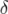model.
• <LESModel>Coeffs: dictionary of coefficients for the respective LESModel, to override the default coefficients.
• <delta>Coeffs: dictionary of coefficients for the delta model.

The LES models used in the tutorials can be listed using foamSearch with the following command. The lists of available models are given in the following sections.

foamSearch \$FOAM_TUTORIALS turbulenceProperties LES.LESModel

#### 7.2.2.1 Incompressible LES turbulence models

For incompressible flows, the LESModel can be chosen from the list below.

DeardorffDiffStress
Differential SGS Stress Equation Model for incompressible flows
Smagorinsky
The Smagorinsky SGS model.
SpalartAllmarasDDES
SpalartAllmaras DDES turbulence model for incompressible flows
SpalartAllmarasDES
SpalartAllmarasDES DES turbulence model for incompressible flows
SpalartAllmarasIDDES
SpalartAllmaras IDDES turbulence model for incompressible flows
WALE
The Wall-adapting local eddy-viscosity (WALE) SGS model.
dynamicKEqn
Dynamic one equation eddy-viscosity model
dynamicLagrangian
Dynamic SGS model with Lagrangian averaging
kEqn
One equation eddy-viscosity model
kOmegaSSTDES
Implementation of the k-omega-SST-DES turbulence model for incompressible flows.

#### 7.2.2.2 Compressible LES turbulence models

For compressible flows, the LESModel can be chosen from the list below.

DeardorffDiffStress
Differential SGS Stress Equation Model for compressible flows
Smagorinsky
The Smagorinsky SGS model.
SpalartAllmarasDDES
SpalartAllmaras DDES turbulence model for compressible flows
SpalartAllmarasDES
SpalartAllmarasDES DES turbulence model for compressible flows
SpalartAllmarasIDDES
SpalartAllmaras IDDES turbulence model for compressible flows
WALE
The Wall-adapting local eddy-viscosity (WALE) SGS model.
dynamicKEqn
Dynamic one equation eddy-viscosity model
dynamicLagrangian
Dynamic SGS model with Lagrangian averaging
kEqn
One equation eddy-viscosity model
kOmegaSSTDES
Implementation of the k-omega-SST-DES turbulence model for compressible flows.

### 7.2.3 Model coefficients

The coefficients for the RAS turbulence models are given default values in their respective source code. If the user wishes to override these default values, then they can do so by adding a sub-dictionary entry to the RAS sub-dictionary file, whose keyword name is that of the model with Coeffs appended, e.g. kEpsilonCoeffs for the kEpsilon model. If the printCoeffs switch is on in the RAS sub-dictionary, an example of the relevant …Coeffs dictionary is printed to standard output when the model is created at the beginning of a run. The user can simply copy this into the RAS sub-dictionary file and edit the entries as required.

### 7.2.4 Wall functions

A range of wall function models is available in OpenFOAM that are applied as boundary conditions on individual patches. This enables different wall function models to be applied to different wall regions. The choice of wall function model is specified through the turbulent viscosity field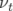in the 0/nut file. Note for OpenFOAM versions prior to v3.0.0: wall functions for compressible RAS are specified through the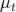field in the 0/mut file, through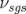in the 0/nuSgs file for incompressible LES and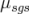in the 0/muSgs file for compressible LES. For example, a 0/nut file:

17
18  dimensions      [0 2 -1 0 0 0 0];
19
20  internalField   uniform 0;
21
22  boundaryField
23  {
24      movingWall
25      {
26          type            nutkWallFunction;
27          value           uniform 0;
28      }
29      fixedWalls
30      {
31          type            nutkWallFunction;
32          value           uniform 0;
33      }
34      frontAndBack
35      {
36          type            empty;
37      }
38  }
39
40
41  // ************************************************************************* //

There are a number of wall function models available in the release, e.g. nutWallFunction, nutRoughWallFunction, nutUSpaldingWallFunction, nutkWallFunction and nutkAtmWallFunction. The user can get the fill list of wall function models using foamInfo:

foamInfo wallFunctions
Within each wall function boundary condition the user can over-ride default settings for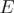,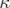and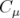through optional E, kappa and Cmu keyword entries.

Having selected the particular wall functions on various patches in the nut/mut file, the user should select epsilonWallFunction on corresponding patches in the epsilon field and kqRwallFunction on corresponding patches in the turbulent fields k, q and R.

OpenFOAM v6 User Guide - 7.2 Turbulence models